Ellipse - Defination

Chapter 10 Class 11 Conic Sections
Concept wise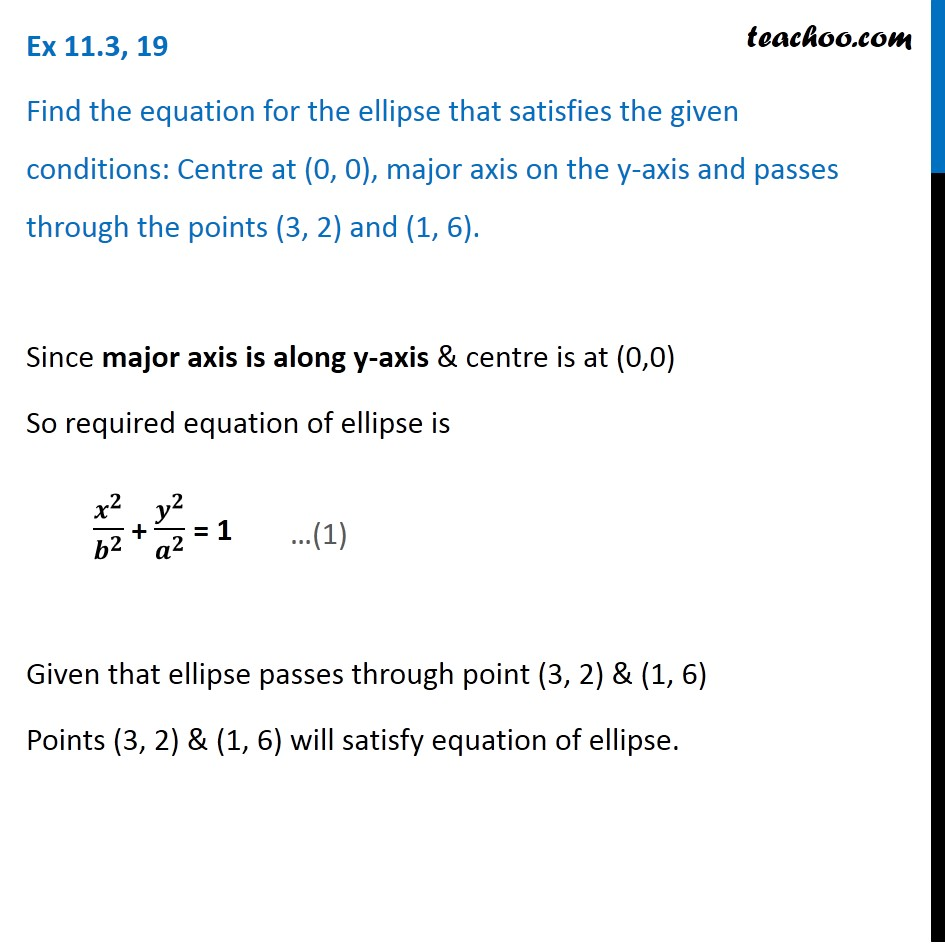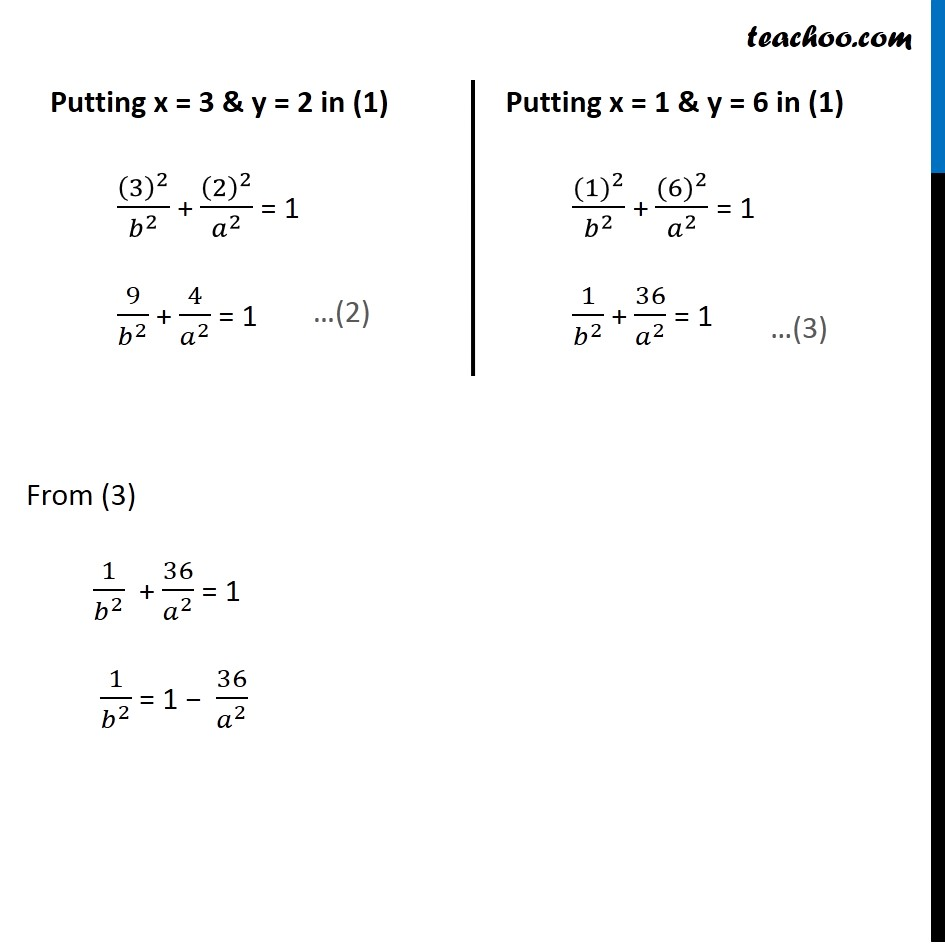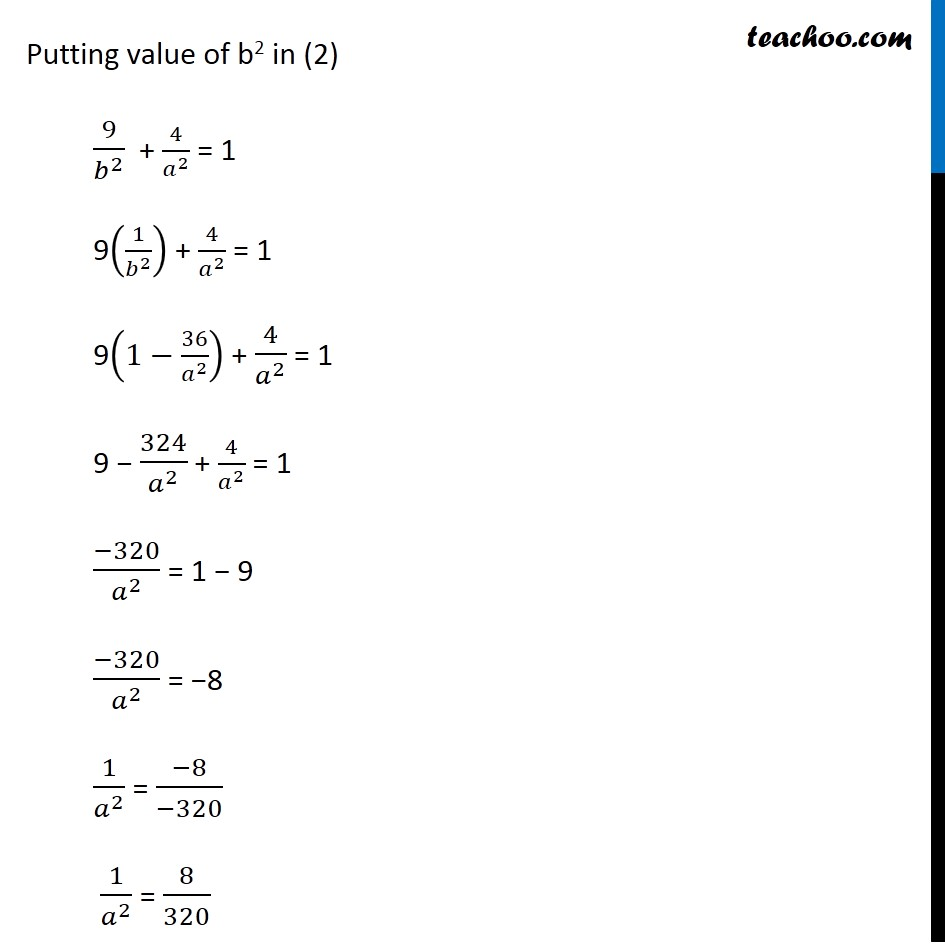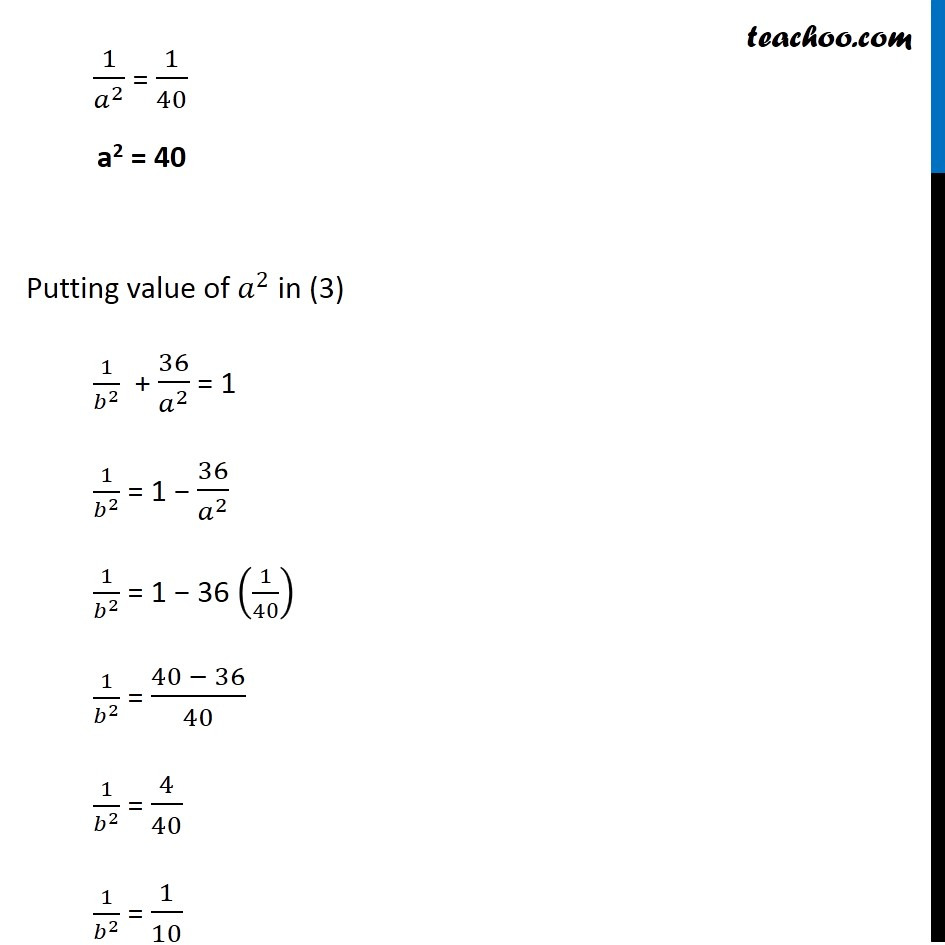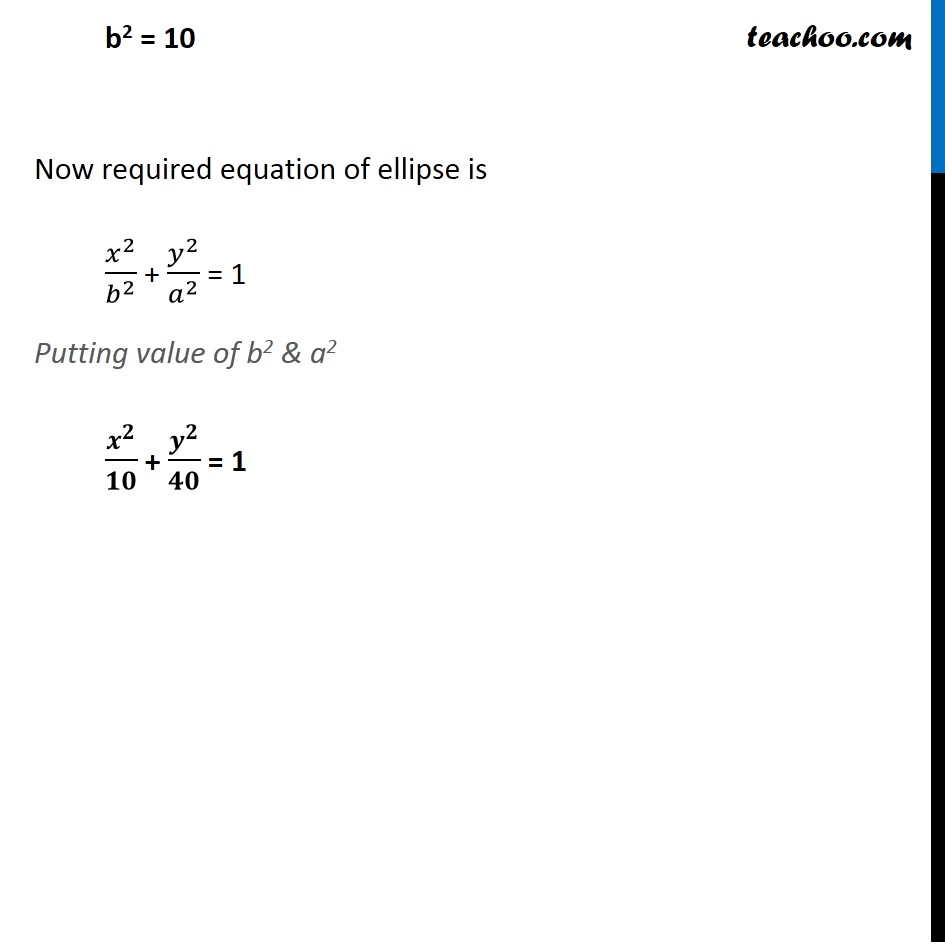Learn in your speed, with individual attention - Teachoo Maths 1-on-1 Class

### Transcript

Ex 10.3, 19 Find the equation for the ellipse that satisfies the given conditions: Centre at (0, 0), major axis on the y-axis and passes through the points (3, 2) and (1, 6). Since major axis is along y-axis & centre is at (0,0) So required equation of ellipse is 𝒙^𝟐/𝒃^𝟐 + 𝒚^𝟐/𝒂^𝟐 = 1 Given that ellipse passes through point (3, 2) & (1, 6) Points (3, 2) & (1, 6) will satisfy equation of ellipse. Putting x = 3 & y = 2 in (1) (3)^2/𝑏^2 + (2)^2/𝑎^2 = 1 9/𝑏^2 + 4/𝑎^2 = 1 Putting x = 1 & y = 6 in (1) 〖(1)〗^2/𝑏^2 + 〖(6)〗^2/𝑎^2 = 1 1/𝑏^2 + 36/𝑎^2 = 1 From (3) 1/𝑏^2 + 36/𝑎^2 = 1 1/𝑏^2 = 1 − 36/𝑎^2 Putting value of b2 in (2) 9/𝑏^2 + 4/𝑎^2 = 1 9(1/𝑏^2 ) + 4/𝑎^2 = 1 9(1−36/𝑎^2 ) + 4/𝑎^2 = 1 9 − 324/𝑎^2 + 4/𝑎^2 = 1 (−320)/𝑎^2 = 1 − 9 (−320)/𝑎^2 = −8 1/𝑎^2 = (−8)/(−320) 1/𝑎^2 = 8/320 1/𝑎^2 = 1/40 a2 = 40 Putting value of 𝑎^2 in (3) 1/𝑏^2 + 36/𝑎^2 = 1 1/𝑏^2 = 1 − 36/𝑎^2 1/𝑏^2 = 1 − 36 (1/40) 1/𝑏^2 = (40 − 36)/40 1/𝑏^2 = 4/40 1/𝑏^2 = 1/10 b2 = 10 Now required equation of ellipse is 𝑥^2/𝑏^2 + 𝑦^2/𝑎^2 = 1 Putting value of b2 & a2 𝒙^𝟐/𝟏𝟎 + 𝒚^𝟐/𝟒𝟎 = 1